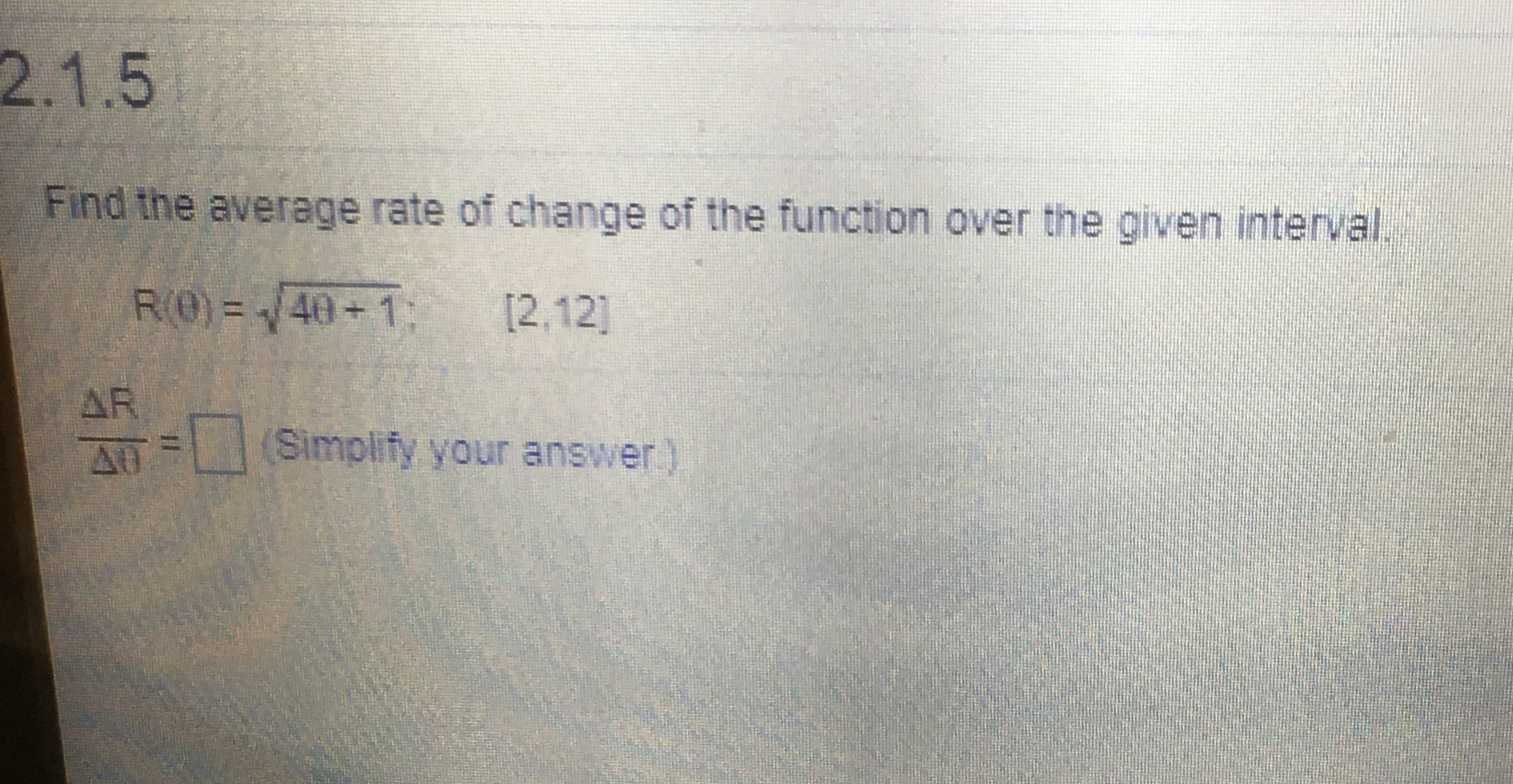# 2.1.5Find the average rate of change of the function over the given interval.R(0) = /40+1:[2,12]AR(Simplify your answer)

Question
10 viewshelp_outlineImage Transcriptionclose2.1.5 Find the average rate of change of the function over the given interval. R(0) = /40+1: [2,12] AR (Simplify your answer) fullscreen
check_circle

Step 1

According to the given information it is required to calculate the average rate of change of the function over the interval:

Step 2

The rate of change of the function f(x) over the interval [a,b] can be calculated as:

Step 3

Now calculate it for the...

### Want to see the full answer?

See Solution

#### Want to see this answer and more?

Solutions are written by subject experts who are available 24/7. Questions are typically answered within 1 hour.*

See Solution
*Response times may vary by subject and question.
Tagged in

### Derivative